Tutorial 5: The ratioPairs Function

The following example shows how to build a complex expression where the top level function, ratioPairs, operates on two groupBy functions nested at the same level.

The ratioPairs functions divide pairs of time series. The first groupBy expression is the numerator and the second groupBy expression is the denominator. Each groupBy function must have the same values. The denominator must have at least one (1) output metric.

Note: The ratioPairs and divideSeries functions each use a custom template, which contains the entire tree structure required for completing the function expression.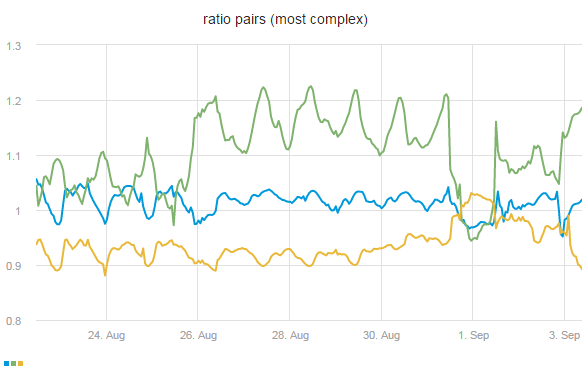How to create a complex function using RatioPairs

1. In the Metrics tab, place the cursor in the blank expression node and click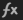.
2. In the Function drop-down list, select ratioPairs.

The template for the ratioPairs function appears with the necessary expression tree structure: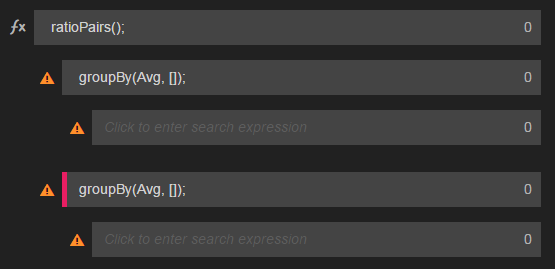Note: You only need to complete the nodes beginning with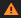.

3. In each groupBy function node:
• In the Aggregation drop-down list, select the Aggregation type: Avg, Sum, Median, Max, Min, Std
• In the GroupBy drop-down list, select the property to group by.
4. In each empty expression node, select property and value pairs, as required.

The Expression Builder now shows both groupBy functions within the ratioPairs function as completed.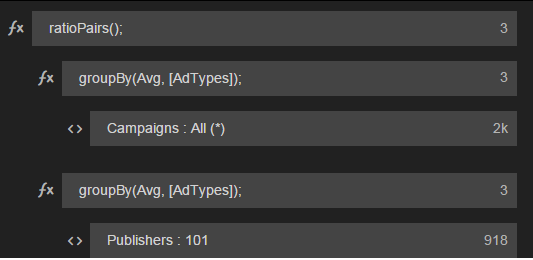5. Click Save. See Metric Expressions.